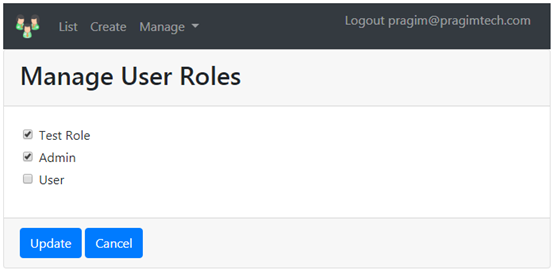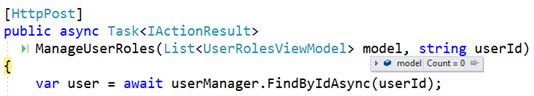Support us .Net Basics C# SQL ASP.NET Aarvi MVC Slides C# Programs Subscribe Download

### Model binding not working on submitting razor view with foreach loop

Suggested Videos
Part 89 - Enforce ON DELETE NO ACTION in entity framework core | Text | Slides

In this video we will discuss one of the common issues we will frequently run into when using asp.net mvc. Why model binding does not work in MVC as expected, if we submit a razor form that contains a foreach loop.

Consider the following ManageUserRoles action methods.

[HttpGet]
{
ViewBag.userId = userId;

var user = await userManager.FindByIdAsync(userId);

if (user == null)
{
ViewBag.ErrorMessage = \$"User with Id = {userId} cannot be found";
return View("NotFound");
}

var model = new List<UserRolesViewModel>();

foreach(var role in roleManager.Roles)
{
var userRolesViewModel = new UserRolesViewModel
{
RoleId = role.Id,
RoleName = role.Name
};

if (await userManager.IsInRoleAsync(user, role.Name))
{
userRolesViewModel.IsSelected = true;
}
else
{
userRolesViewModel.IsSelected = false;
}

}

return View(model);
}

[HttpPost]
ManageUserRoles(List<UserRolesViewModel> model, string userId)
{
var user = await userManager.FindByIdAsync(userId);

if (user == null)
{
ViewBag.ErrorMessage = \$"User with Id = {userId} cannot be found";
return View("NotFound");
}

var roles = await userManager.GetRolesAsync(user);
var result = await userManager.RemoveFromRolesAsync(user, roles);

if (!result.Succeeded)
{
ModelState.AddModelError("", "Cannot remove user existing roles");
return View(model);
}

model.Where(x => x.IsSelected).Select(y => y.RoleName));

if (!result.Succeeded)
{
return View(model);
}

return RedirectToAction("EditUser", new { Id = userId });

}

for loop in razor form

@for (int i = 0; i < Model.Count; i++)
{
<div class="form-check m-1">
<input type="hidden" asp-for="@Model[i].RoleId" />
<input type="hidden" asp-for="@Model[i].RoleName" />
<input asp-for="@Model[i].IsSelected" class="form-check-input" />
<label class="form-check-label" asp-for="@Model[i].IsSelected">
@Model[i].RoleName
</label>
</div>
}
• The model for the view is List<UserRolesViewModel>
• We are using a for loop to loop through each UserRolesViewModel object
• The required input elements are dynamically generated
• The view looks as shown below.The following is the HTML of the generated input elements.

<div class="form-check m-1">
<input type="hidden" id="z0__RoleId" name=".RoleId" value="4defae7d-6392-4a5d-b257-53b852037219" />
<input type="hidden" id="z0__RoleName" name=".RoleName" value="Test Role" />
<input class="form-check-input" type="checkbox" data-val="true" id="z0__IsSelected" name=".IsSelected" value="true" />
<label class="form-check-label" for="z0__IsSelected">
Test Role
</label>
</div>
<div class="form-check m-1">
<input type="hidden" id="z1__RoleId" name=".RoleId" value="60fce30e-452d-49af-986f-5736515d6897" />
<input type="hidden" id="z1__RoleName" name=".RoleName" value="Admin" />
<input class="form-check-input" type="checkbox" checked="checked" id="z1__IsSelected" name=".IsSelected" value="true" />
<label class="form-check-label" for="z1__IsSelected">
</label>
</div>
<div class="form-check m-1">
<input type="hidden" id="z2__RoleId" name=".RoleId" value="e23ac65d-b1cc-483a-bde8-83e1970bbaf1" />
<input type="hidden" id="z2__RoleName" name=".RoleName" value="User" />
<input class="form-check-input" type="checkbox" data-val="true" id="z2__IsSelected" name=".IsSelected" value="true" />
<label class="form-check-label" for="z2__IsSelected">
User
</label>
</div>

Notice, the name attribute of the input elements. They have an integer indexer like the following, so the model binder in asp.net mvc knows it has to map these values to the list parameter on the controller action.
• name=".RoleId"
• name=".RoleId"
• name=".RoleId"
When this form is submitted, the values in these input elements are automatically mapped by the model binder to the List parameter on the controller action. Notice, in this example we have 3 elements in the list.foreach loop in razor form

What happens if we use a foreach loop instead of a for loop in the razor form.

@foreach (var role in Model)
{
<div class="form-check m-1">
<input type="hidden" asp-for="@role.RoleId" />
<input type="hidden" asp-for="@role.RoleName" />
<input asp-for="@role.IsSelected" class="form-check-input" />
<label class="form-check-label" asp-for="@role.IsSelected">
@role.RoleName
</label>
</div>
}

Model binder in asp.net mvc does not bind the values from the input elements to the list parameter on the controller action. Notice in this case we have ZERO elements in the list.Why did model binding fail when we use foreach loop

The following is the generated HTML when we use a foreach loop. Notice the name attribute of the input elements. They are not unique and there is no integer indexer, so the model binder does not know it has to bind the values from these input elements to the list parameter on the controller action. This is the reason the list parameter on the controller action is empty.

<div class="form-check m-1">
<input type="hidden" id="role_RoleId" name="role.RoleId" value="4defae7d-6392-4a5d-b257-53b852037219" />
<input type="hidden" id="role_RoleName" name="role.RoleName" value="Test Role" />
<input class="form-check-input" type="checkbox" data-val="true" id="role_IsSelected" name="role.IsSelected" value="true" />
<label class="form-check-label" for="role_IsSelected">
Test Role
</label>
</div>
<div class="form-check m-1">
<input type="hidden" id="role_RoleId" name="role.RoleId" value="60fce30e-452d-49af-986f-5736515d6897" />
<input type="hidden" id="role_RoleName" name="role.RoleName" value="Admin" />
<input class="form-check-input" type="checkbox" id="role_IsSelected" name="role.IsSelected" value="true" />
<label class="form-check-label" for="role_IsSelected">
</label>
</div>
<div class="form-check m-1">
<input type="hidden" id="role_RoleId" name="role.RoleId" value="e23ac65d-b1cc-483a-bde8-83e1970bbaf1" />
<input type="hidden" id="role_RoleName" name="role.RoleName" value="User" />
<input class="form-check-input" type="checkbox" id="role_IsSelected" name="role.IsSelected" value="true" />
<label class="form-check-label" for="role_IsSelected">
User
</label>
</div>

Also notice, the ids of the checkboxes are not unique. The for attribute value of the labels are also not unique. As a result, when we click on the labels, only the first checkbox gets checked or unchecked.

For the ID and Name attribute values to be unique, use a for loop instead of a foreach loop. Hope this helps you save sometime if you inadvertently run into this issue.